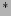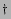Next: Introduction

# Joint statistics of amplitudes and phases in Wave Turbulence Physica D, 201, 121, 2005.

Yeontaek Choi, Yuri V. Lvovand Sergey NazarenkoMathematics Institute, The University of Warwick, Coventry, CV4 7AL, UKDepartment of Mathematical Sciences, Rensselaer Polytechnic Institute, Troy, NY 12180

### Abstract:

Random Phase Approximation (RPA) provides a very convenient tool to study the ensembles of weakly interacting waves, commonly called Wave Turbulence. In its traditional formulation, RPA assumes that phases of interacting waves are random quantities but it usually ignores randomness of their amplitudes. Recently, RPA was generalised in a way that takes into account the amplitude randomness and it was applied to study of the higher momenta and probability densities of wave amplitudes. However, to have a meaningful description of wave turbulence the RPA properties assumed for the initial fields must be proven to survive over the nonlinear evolution time, and such a proof is the main goal of the present paper. We derive an evolution equation for the full probability density function which contains the complete information about the joint statistics of all wave amplitudes and phases. We show that, for any initial statistics of the amplitudes, the phase factors remain statistically independent uniformly distributed variables. If in addition the initial amplitudes are also independent variables (but with arbitrary distributions) they will remain independent when considered in small sets which are much less than the total number of modes. However, if the size of a set is of order of the total number of modes then the joint probability density for this set is not factorisable into the product of one-mode probabilities. In the other words, the modes in such a set are involved in a collective'' (correlated) motion. We also study new type of correlators describing the phase statistics.Next: Introduction
Dr Yuri V Lvov 2007-01-17